# Introduction

Mathematics is broadly divided into two major Parts:

1. Discrete mathematics
2. Continuous mathematics

# Discrete mathematics

In discrete mathematics, the number system is usually integer while in a continuous mathematics number system is usually real numbers.

Integer means multiple of some basic unit and real numbers means all the possible values. e.g. 1 and 2 is an integer while all the possible values between 1 and 2 are the real numbers.

As a result, the discrete variable cannot become infinitely small, and as a concept like the instantaneous rate of change is not available which is the basic concept in continuous mathematics.

# Definition

Discrete mathematics is the branch of mathematics devoted to the study of the discrete object that uses arithmetic and algebra in contrast to other branches whose main concern is with a continuous function.

# Examples:

• A drawer contains 10 black and 10 white socks. What is the least number of socks one must pull out to be sure to get a matched pair?
• How can a circuit that adds two integers and a circuit that compares two integers be designed?
• A Variable name in programming language must be either a letter or a letter followed by a decimal digit. How many different variable names are there in this language?
• A computer system consists of five subsystems. Each subsystem might fail independently with a probability of 0.2. The failure of any subsystem will lead to the failure of the whole computer system. Given that the computer system fails, what is the probability that system one and only subsystem one fails?

# Importance of discrete mathematics

Discrete mathematics is a rapidly growing and increasingly used area of mathematics with many practical and relevant applications. It is an excellent tool for improving reasoning and problem-solving skills and is appropriate for all at all levels and of all abilities.

The importance of discrete mathematics lies in the fact that discrete mathematics deals with sets and not numbers.

This growth of non-numerical mathematics and from the point of view of practical application discrete mathematics has taken an important place in today's mathematics.

Advances in Computer technology have given discrete mathematics a big boost. A key reason for the importance of discrete mathematics is that information is stored and manipulated by computing machine in a discrete fashion. thus discrete mathematics is fundamental to the Science and Technology specific to the computer age.

--

--

--

## More from Bitu

Love podcasts or audiobooks? Learn on the go with our new app.

## Finding best strategy in Casino roulette## Fibonacci Numbers and Algorithms to Compute Them — Part 1## Probability theory: Geometric distribution## Analysis of Algorithms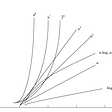## My New Favorite Math Book Helps Everyone See the Beauty and Humanity of Math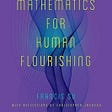## Covariance and Correlation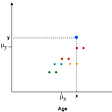## Differential Equations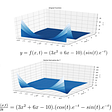## A Visual Introduction to Einstein Notation and why you should Learn Tensor Calculus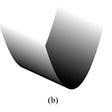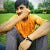## Paulette Chaffee Offers These 5 Tips to Help Kids Love Math## Calculus 101